For a full list of BASHing data blog posts, see the index page.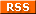# How to find distances between lat/lons for geochecking

Geochecking can be slow and fiddly. One useful strategy is to calculate the difference between latitude/longitude (lat/lon) as provided by the data owner, and as listed for that location in a reference such as a gazetteer. If the difference is large, there's a problem.

Here I explain how to use AWK to calculate the differences for a table-ful of lat/lons. For demonstration purposes, I'll use the tab-separated table "demo" (below). Imagine that the "LatData" and "LonData" figures are the provided ones to be checked, and that the "LatRef" and "LonRef" figures are reference ones I found for the sites using Google Maps, based on verbal descriptions of the sites (not shown):

 Site LatData LonData LatRef LonRef 1 -42.1333 145.8833 -42.1364 145.8772 2 -41.6500 145.0833 -41.5467 145.1640 3 -41.6667 147.7500 -41.6327 147.7615 4 -41.2000 147.9000 -41.2306 147.8892 5 -40.9500 145.2500 -40.9486 145.2478 6 -42.9833 147.9500 -42.9708 147.9653 7 -42.1167 145.7667 -42.1185 145.7743 8 -41.0500 144.6500 -41.1725 145.4778 9 -42.7167 147.7500 -42.7089 147.6839 10 -41.7833 146.9333 -41.8039 146.9556

What I'll do is calculate for each site the Euclidean distance (or "Pythagorean distance") between data and reference lat/lons. This distance isn't as accurate as the great circle distance between the points, but it's good enough if the points are close together (see bottom of this page), and in any case I'm looking for unusually big distances between provided and reference lat/lons.

How big is "big"? It depends in part in how accurate the lat/lons are claimed to be. In the "demo" table, it looks like the lat/lons were converted from DMS to the nearest 1 minute. For example, 145.0833 is 145°05' E, and -42.7167 is 42°43' S. Since 1 minute is roughly 1.9 km in latitude, my arbitrary threshold for "bigness" in distance will be 2 km. If the distance between data and reference lat/lons is 2 km or greater, I'll suspect that something's wrong.

Below is the AWK command I'll use. It's built up step-by-step in the notes towards the bottom of this page, and also saved as a function.

awk -F"\t" 'BEGIN {pi=3.14159} NR==1 {print \$0 FS "Diff"} NR>1 {printf("%s\t%.1f\n",\$0,sqrt(((\$4-\$2)*111.32)^2 + ((\$5-\$3)*111.32*cos(\$4*(pi/180)))^2))}' demo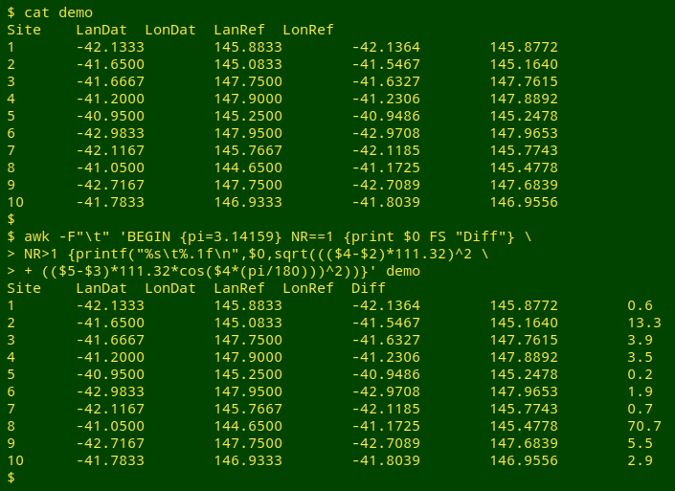Hmmm. There could be problems with the lat/lons for sites 2, 3,4, 8, 9 and 10, especially sites 2 and 8! (See below for a shorthand function for the complicated command.)

Building the command. Calculating a Euclidean or Pythagorean distance between two locations is a little bit like calculating the length of the hypotenuse of a right triangle: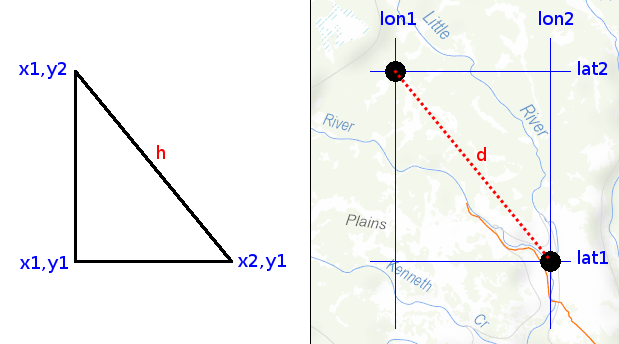The length of the hypotenuse is the square root of the sum of the squares of the lengths of the two shorter sides:

h = √((y2-y1)2 + (x2-x1)2)

Calculating the distance between 2 latitudes in decimal degrees is simple, because 1 degree of latitude is about 111.32 km, and over small-ish distances on the surface of the Earth, the latitude difference expressed as a distance in km is:

(lat2 - lat1) * 111.32

However, the distance between 2 longitudes in decimal degrees is not simply the longitude difference times 111.32 km, because the distance between any two longitudes varies with latitude. The distance is greatest at the Equator, and smallest near the Poles, and it varies with the cosine of the latitude. If the latitudes are close together, it doesn't much matter which one is chosen to adjust the distance (see bottom of page):

(lon2 - lon1) * 111.32 * cos(lat2)

Translating what we've got so far into AWK, the code is:

(lat2-lat1)*111.32     # latitude distance

((lat2-lat1)*111.32)^2     # latitude distance squared

(lon2-lon1)*111.32*cos(lat2)     # longitude distance

((lon2-lon1)*111.32*cos(lat2))^2     # longitude distance squared

((lat2-lat1)*111.32)^2 + ((lon2-lon1)*111.32*cos(lat2))^2
# sum of the squares

sqrt(((lat2-lat1)*111.32)^2 + ((lon2-lon1)*111.32*cos(lat2))^2)
# square root of the sum of the squares

Unfortunately, this code won't work properly, because the cos built-in function in AWK takes angles in radians, not degrees. To convert degrees to radians:

Modifying the code:

sqrt(((lat2-lat1)*111.32)^2 + ((lon2-lon1)*111.32*cos(lat2*(pi/180)))^2)

I've written "pi" there because AWK doesn't know the constant π. In the command, I'll define "pi" as 3.14159 in a BEGIN statement.

BEGIN {pi=3.14159}

To print the calculated distance in km to 1 decimal place I'll use AWK's printf:

printf("%.1f\n",sqrt(((lat2-lat1)*111.32)^2 + ((lon2-lon1)*111.32*cos(lat2*(pi/180)))^2))

And to print the whole line with the distance added as a new, tab-separated field:

printf("%s\t%.1f\n",\$0,sqrt(((lat2-lat1)*111.32)^2 + ((lon2-lon1)*111.32*cos(lat2*(pi/180)))^2))

Nearly done. To print just the first line of the table with an added field for the distance difference, I'll include:

NR==1 {print \$0 FS "Diff"}

And to run the calculation on the remaining lines:

NR>1 {printf("%s\t%.1f\n",\$0,sqrt(((lat2-lat1)*111.32)^2 + ((lon2-lon1)*111.32*cos(lat2*(pi/180)))^2))}

Putting it all together, I get the command used above. This command has far too many round brackets to keep track of, so I'll save the command as a function, "latlond". The function will take 5 arguments: filename, field with lat1, field with lon1, field with lat2 and field with lon2. For ease of remembering, "lat1" and "lon1" will be the provided lat/lon, and "lat2" and "lon2" will be the reference lat/lon. Here's the function:

latlond() { awk -F"\t" -v lat1="\$2" -v lon1="\$3" -v lat2="\$4" -v lon2="\$5" 'BEGIN {pi=3.14159} NR==1 {print \$0 FS "Diff"} NR>1 {printf("%s\t%.1f\n",\$0,sqrt(((\$lat2-\$lat1)*111.32)^2 + ((\$lon2-\$lon1)*111.32*cos(\$lat2*(pi/180)))^2))}' "\$1"; }

And here's the function at work on "demo":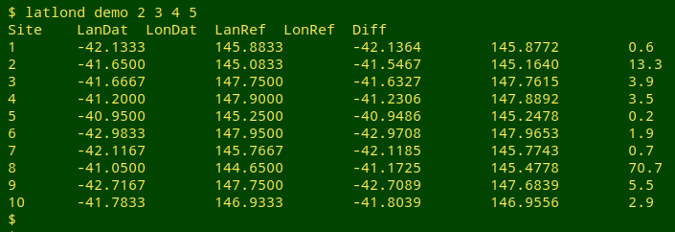The Euclidean approximation. For the "demo" table, the Euclidean and great circle distances are identical to the nearest 0.1 km. The 10 sites were checked online with the Haversine formula for great circle distances.

Latitude to use for longitude distance correction. There's only a small difference, and only at the biggest distance, between using the provided latitude and the reference latitude for the longitude distance correction in the "demo" table: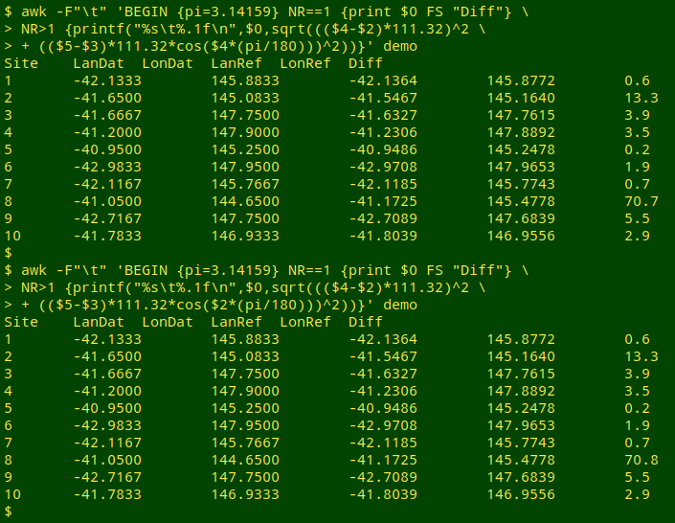Last update: 2020-01-13
The blog posts on this website are licensed under a